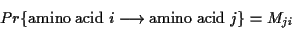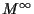Next: PAM distance. Up: Definitions Previous: Definitions

#### Mutation model.

The evolution between sequences can be modelled by mutation matrices. A mutation matrix, denoted by M, describes the probabilities of amino acid mutations for a given period of evolution.This corresponds to a model of evolution in which amino acids mutate randomly and independently from one another but according to some predefined probabilities depending on the amino acid itself. This is a Markovian model of evolution and while simple, it is one of the best models.

Intrinsic properties of amino acids, like hydrophobicity, size, charge, etc. can be modelled by appropriate mutation matrices. Dependencies which relate one amino acid characteristic to the characteristics of its neighbours are not possible to model through this mechanism.

Amino acids appear in nature with different frequencies. These frequencies are denoted by fi and correspond to the steady state of the Markov process defined by the matrix M. I.e. the vector f is any of the columns ofor the eigenvector of M whose corresponding eigenvalue is 1 (Mf=f).

This model of evolution is symmetric, i.e. the probability of having an i which mutates to a jis the same as starting with a j which mutates into an i. This symmetry means

fi Mji = fj MijNext: PAM distance. Up: Definitions Previous: Definitions
Gaston Gonnet
1998-07-14